# Jeopardy Surface Area JEOPARDY Prisms Cylinders Pyramids Cones

• Slides: 52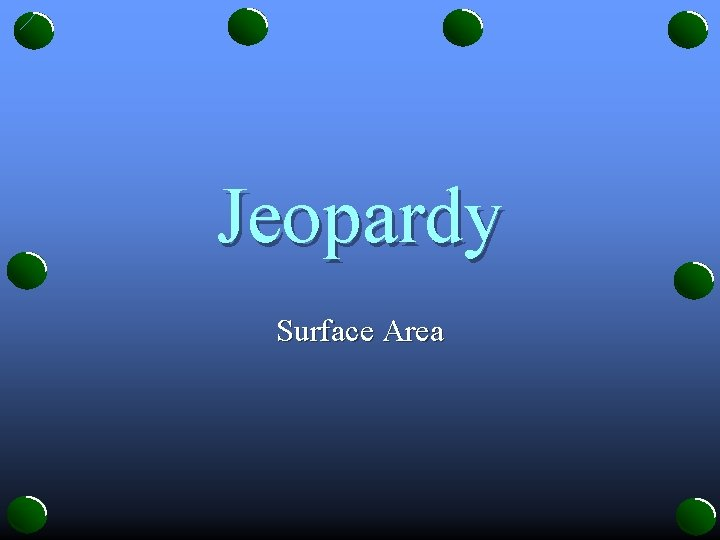Jeopardy Surface Area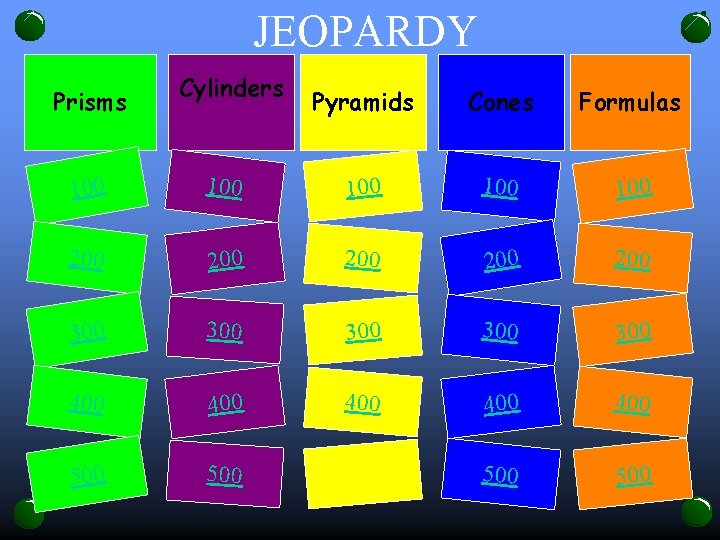JEOPARDY Prisms Cylinders Pyramids Cones Formulas 100 100 100 200 200 200 300 300 300 400 400 400 500 500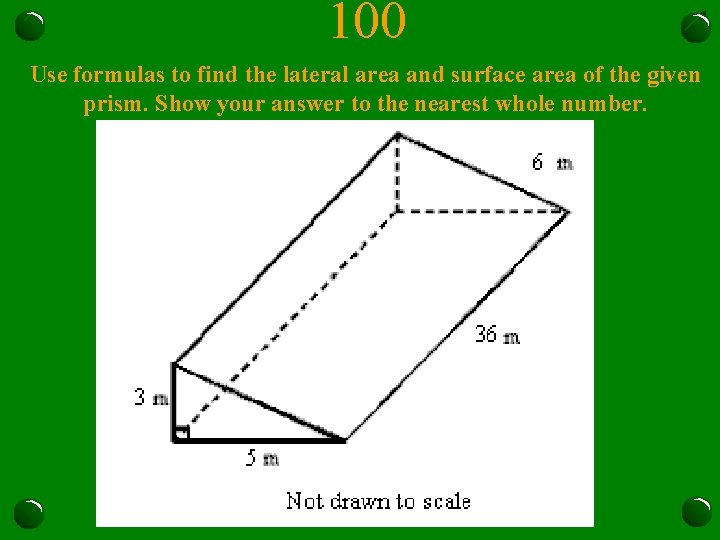100 Use formulas to find the lateral area and surface area of the given prism. Show your answer to the nearest whole number.Jeopardy200 Use formulas to find the lateral area and surface area of the given prism. Show your answer to the nearest whole number.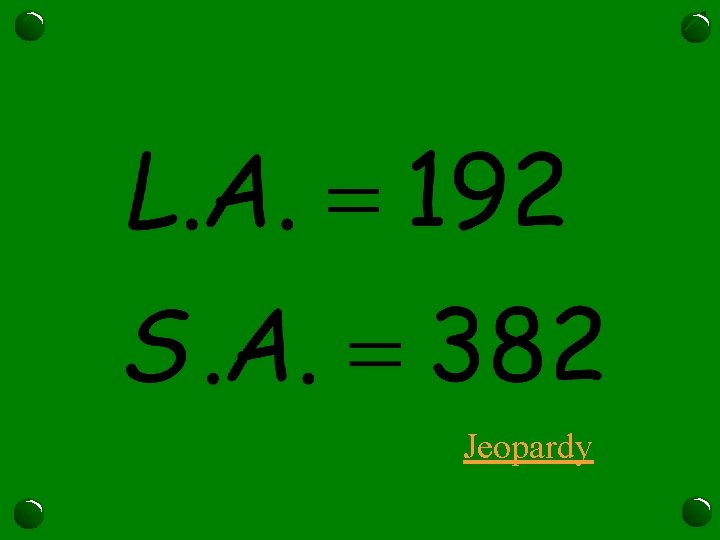Jeopardy300 A jewelry store buys small boxes in which to wrap items that they sell. The diagram below shows one of the boxes. Find the lateral area and the surface area of the box to the nearest whole number.Jeopardy400 Use formulas to find the lateral area and surface area of the given prism. Show your answer to the nearest whole number.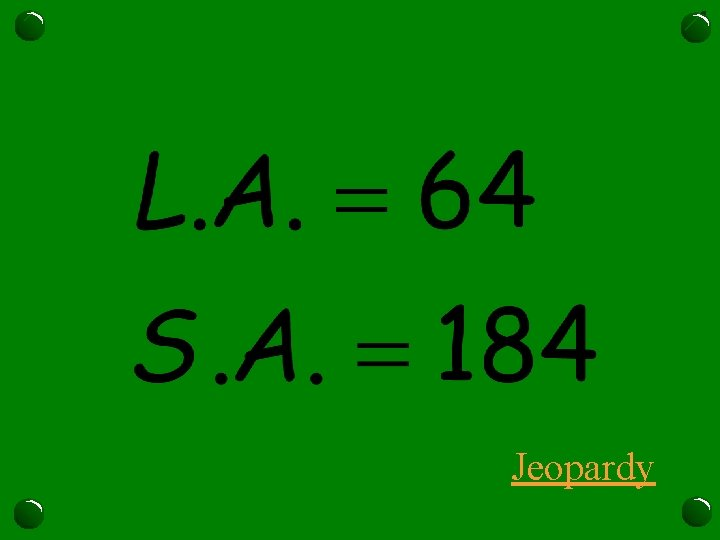Jeopardy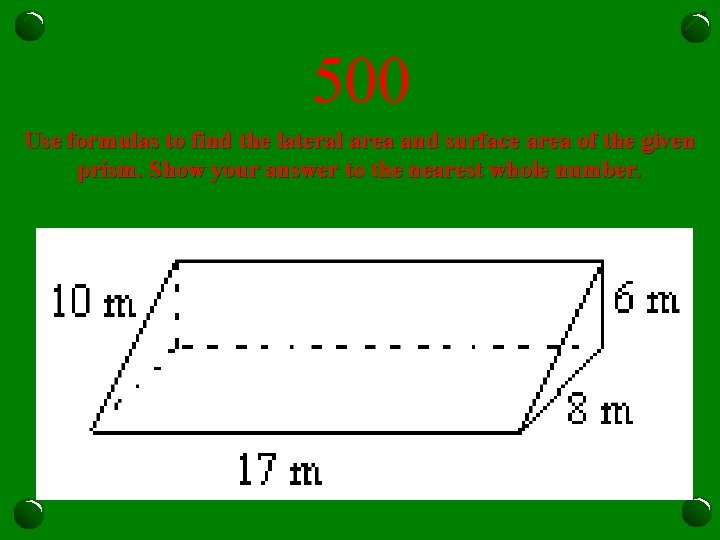500 Use formulas to find the lateral area and surface area of the given prism. Show your answer to the nearest whole number.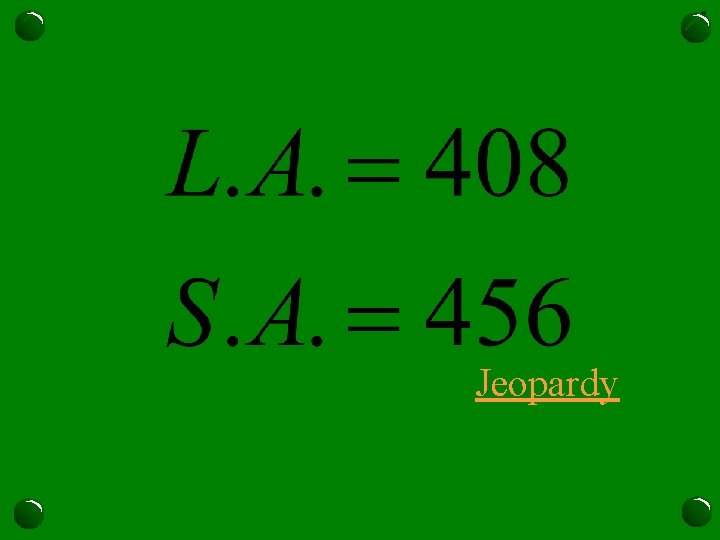Jeopardy100 Find the surface area of the cylinder in terms of pi.Jeopardy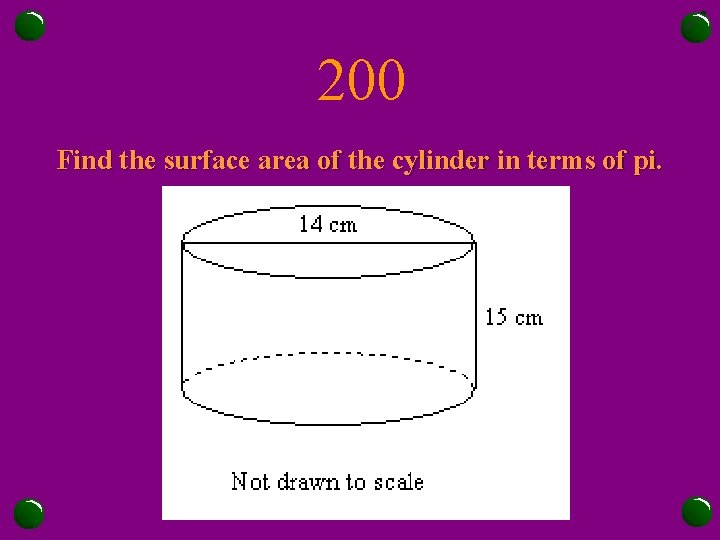200 Find the surface area of the cylinder in terms of pi.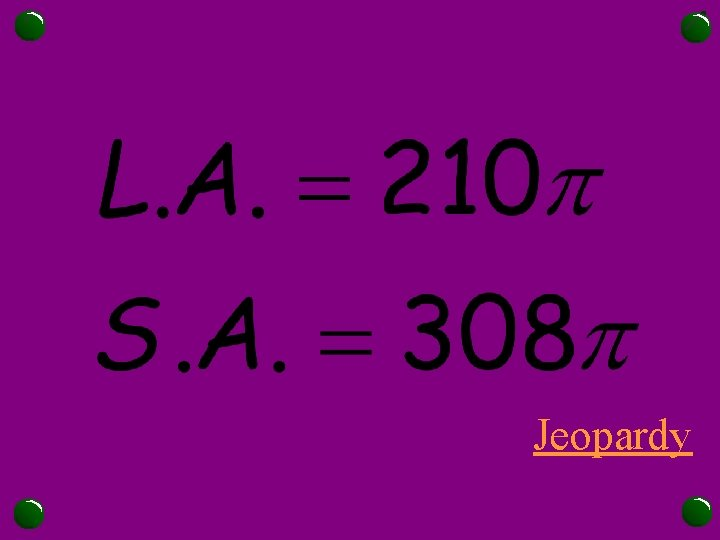Jeopardy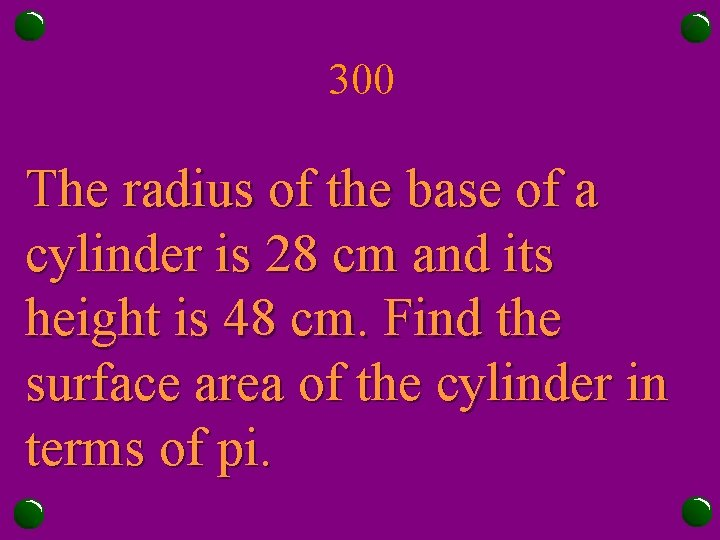300 The radius of the base of a cylinder is 28 cm and its height is 48 cm. Find the surface area of the cylinder in terms of pi.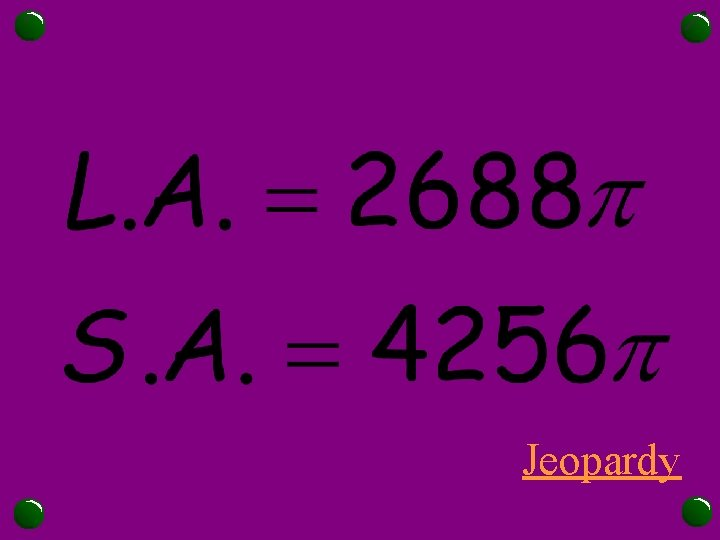Jeopardy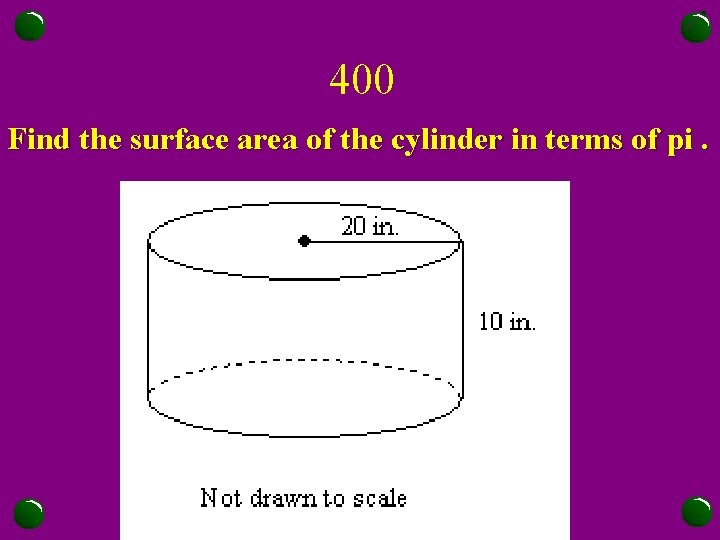400 Find the surface area of the cylinder in terms of pi.Jeopardy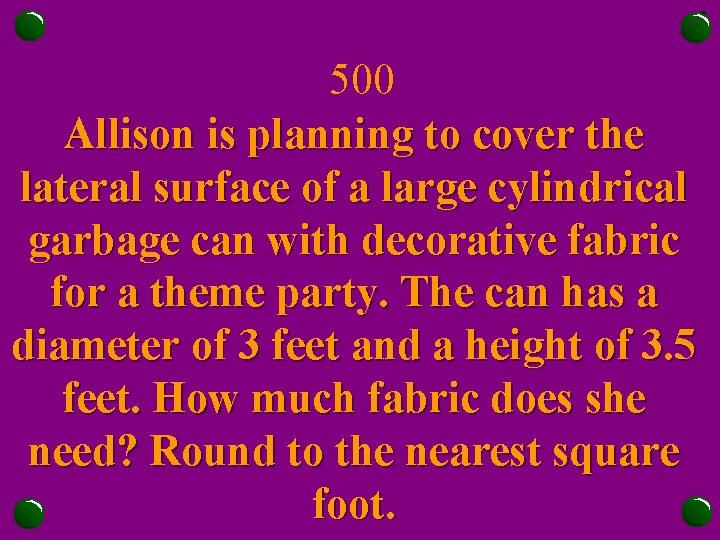500 Allison is planning to cover the lateral surface of a large cylindrical garbage can with decorative fabric for a theme party. The can has a diameter of 3 feet and a height of 3. 5 feet. How much fabric does she need? Round to the nearest square foot.Jeopardy100 Find the surface area of the pyramid shown to the nearest whole number.Jeopardy200 Find the surface area of the pyramid shown to the nearest whole number.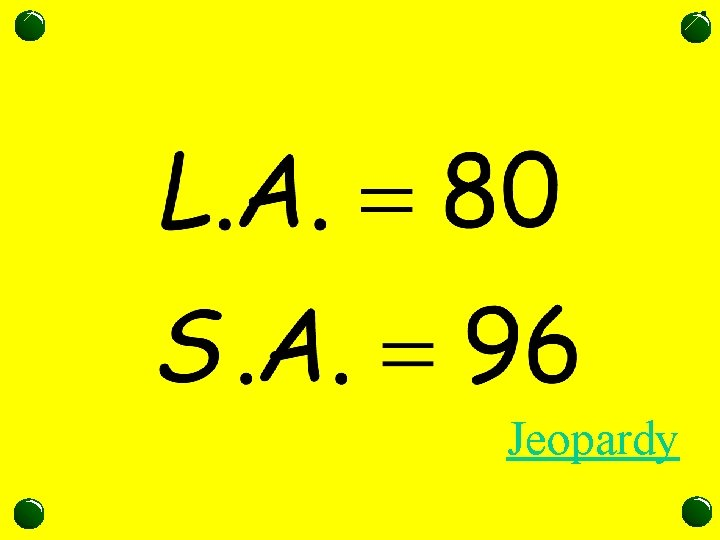Jeopardy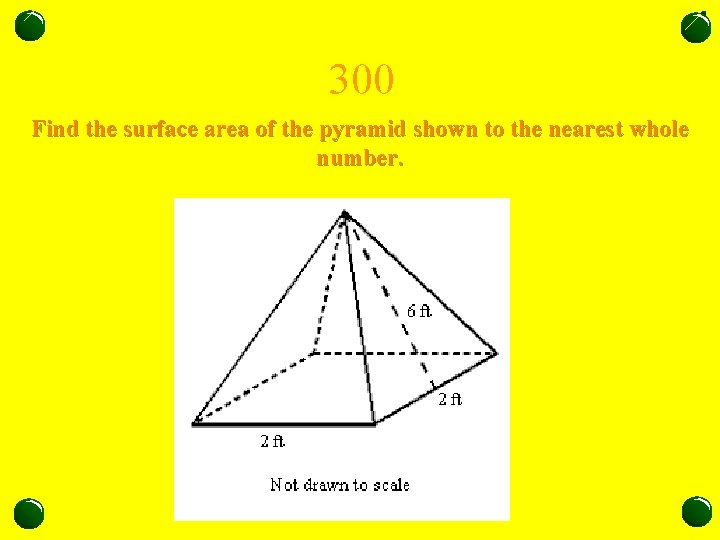300 Find the surface area of the pyramid shown to the nearest whole number.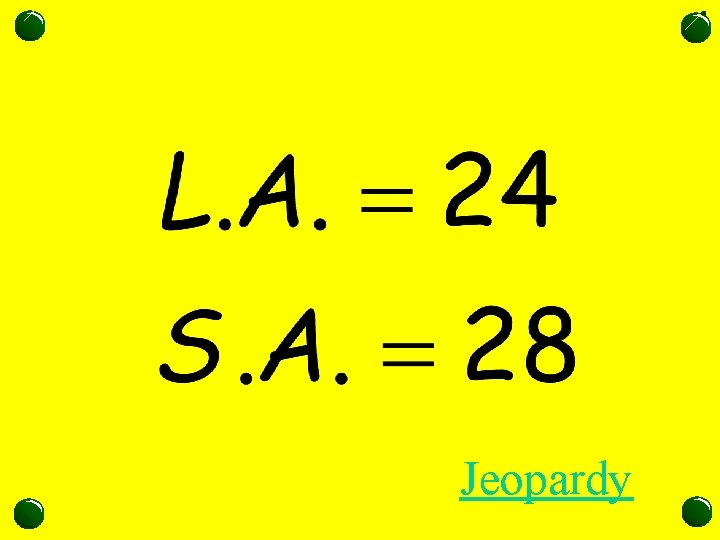Jeopardy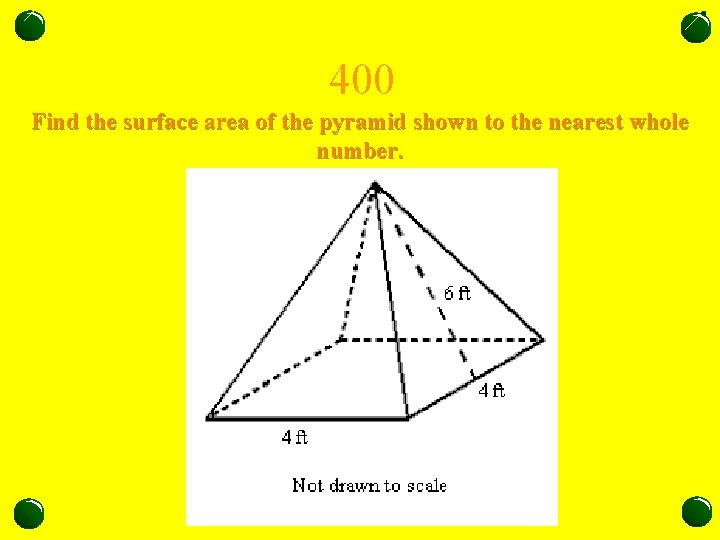400 Find the surface area of the pyramid shown to the nearest whole number.Jeopardy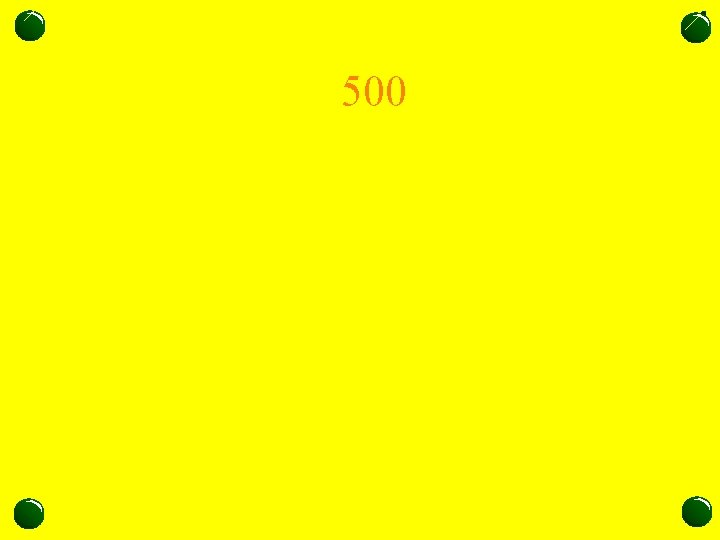500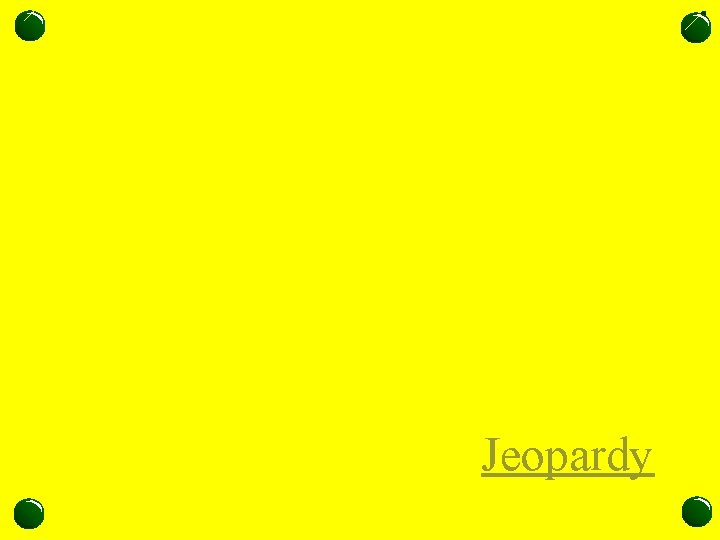Jeopardy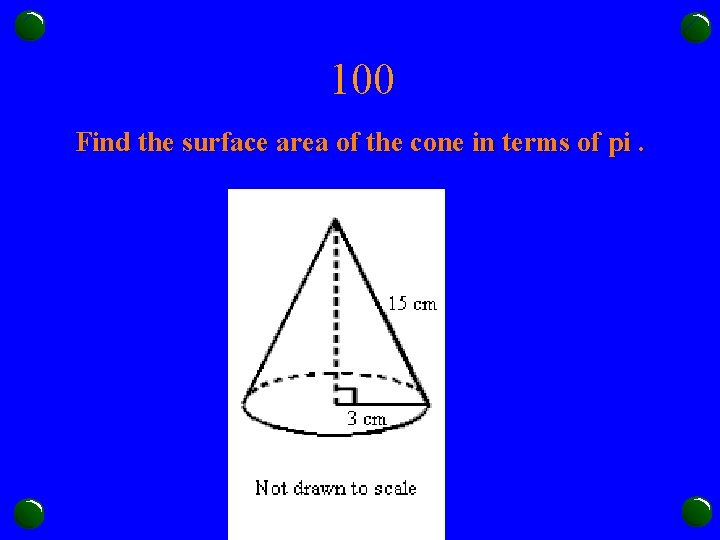100 Find the surface area of the cone in terms of pi.Jeopardy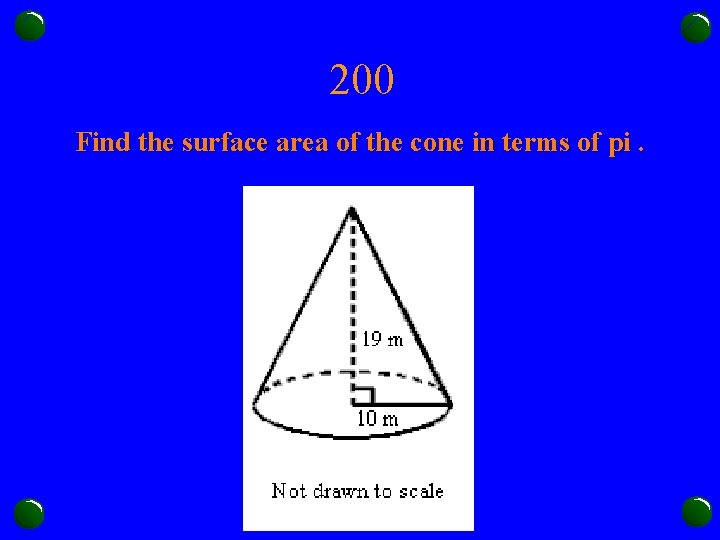200 Find the surface area of the cone in terms of pi.Jeopardy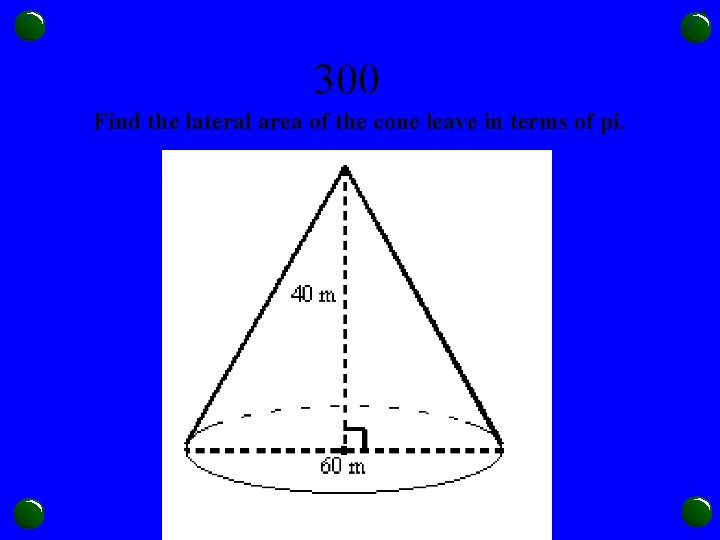300 Find the lateral area of the cone leave in terms of pi.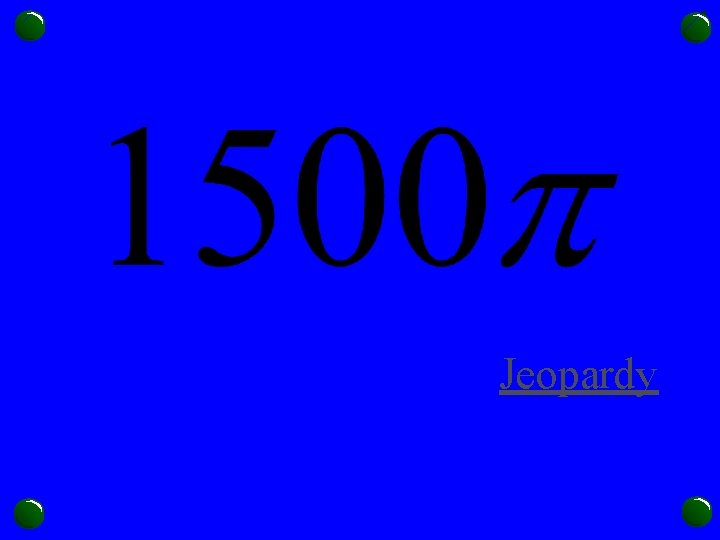Jeopardy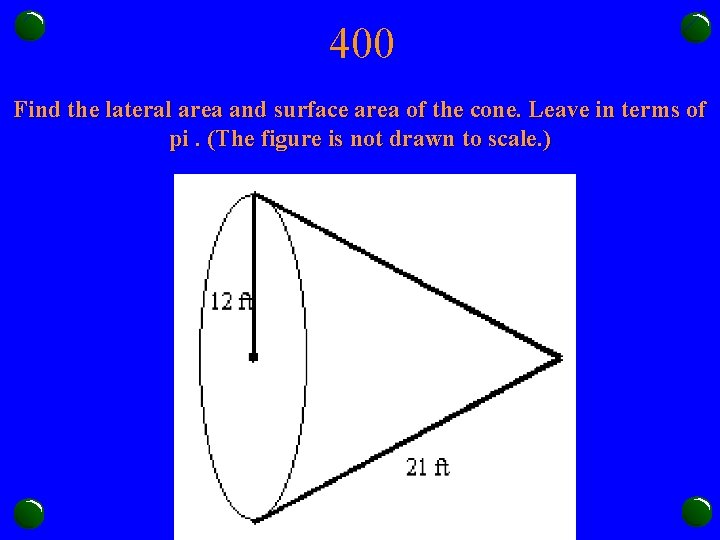400 Find the lateral area and surface area of the cone. Leave in terms of pi. (The figure is not drawn to scale. )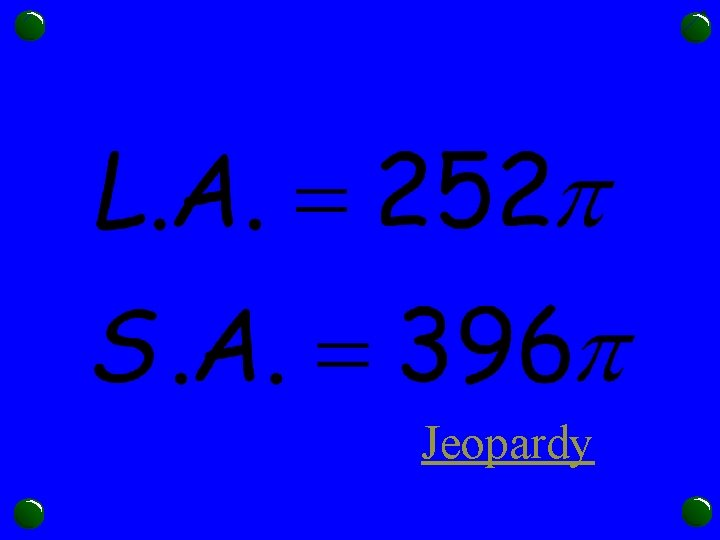Jeopardy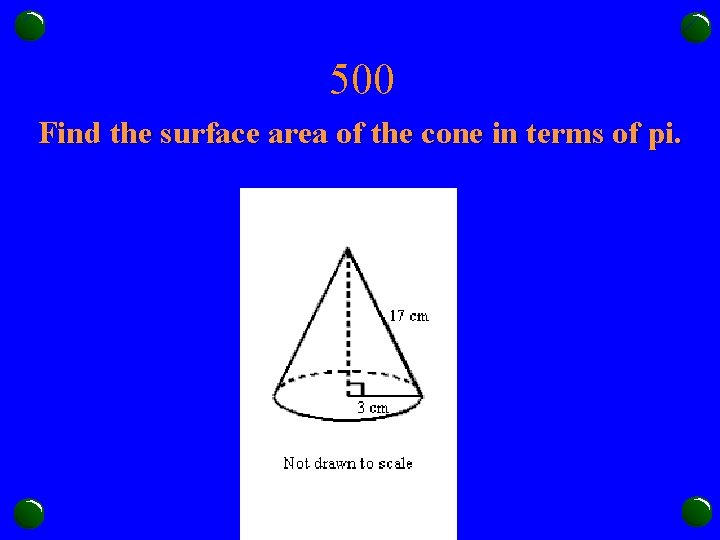500 Find the surface area of the cone in terms of pi.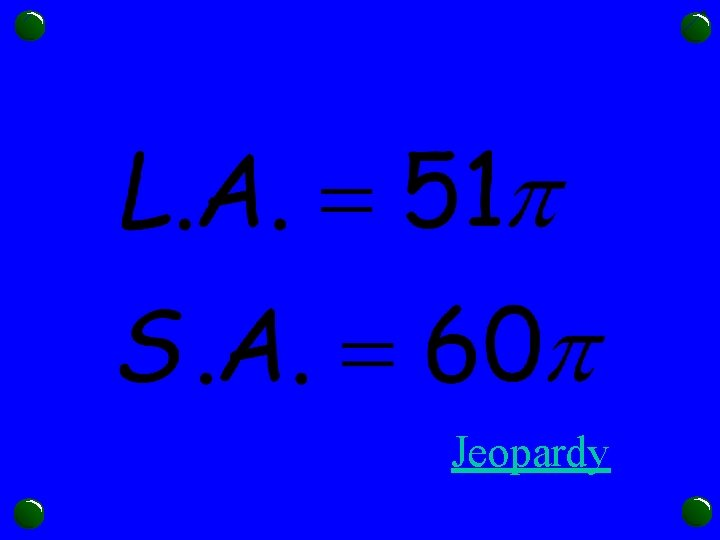Jeopardy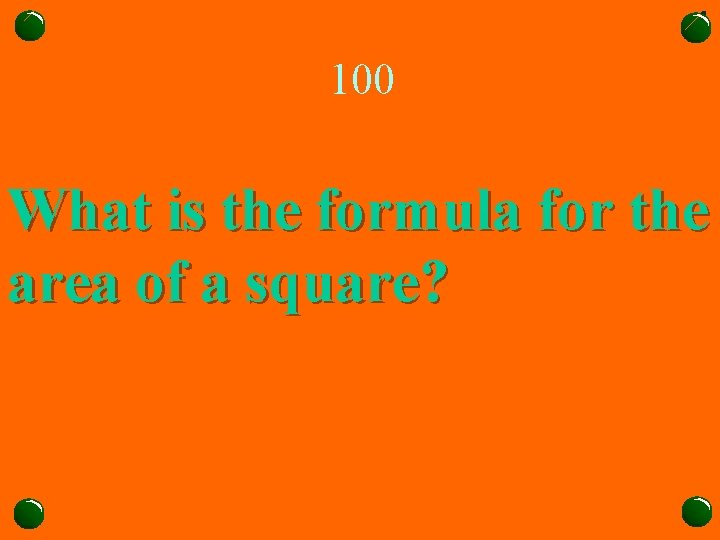100 What is the formula for the area of a square?Jeopardy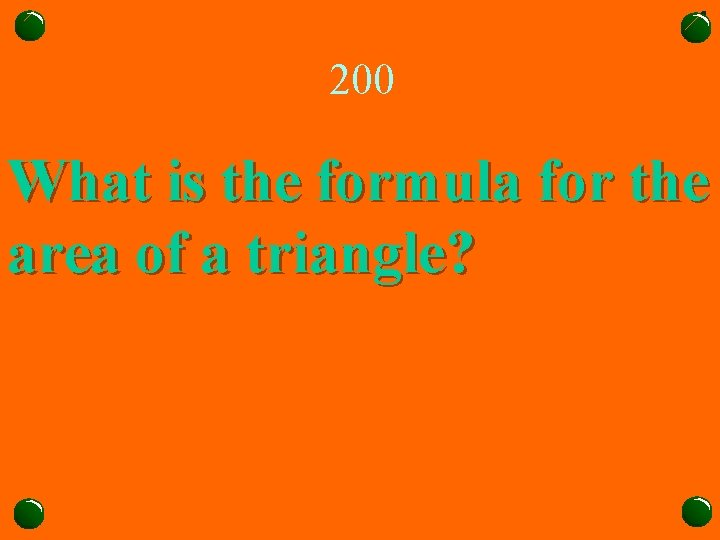200 What is the formula for the area of a triangle?Jeopardy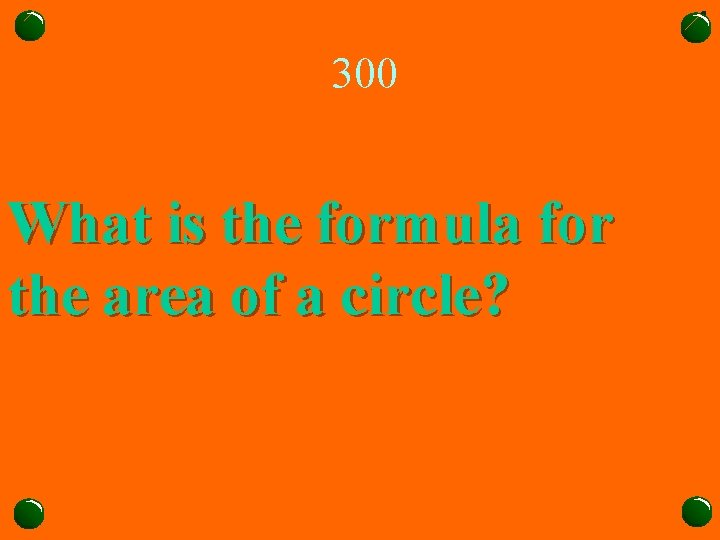300 What is the formula for the area of a circle?Jeopardy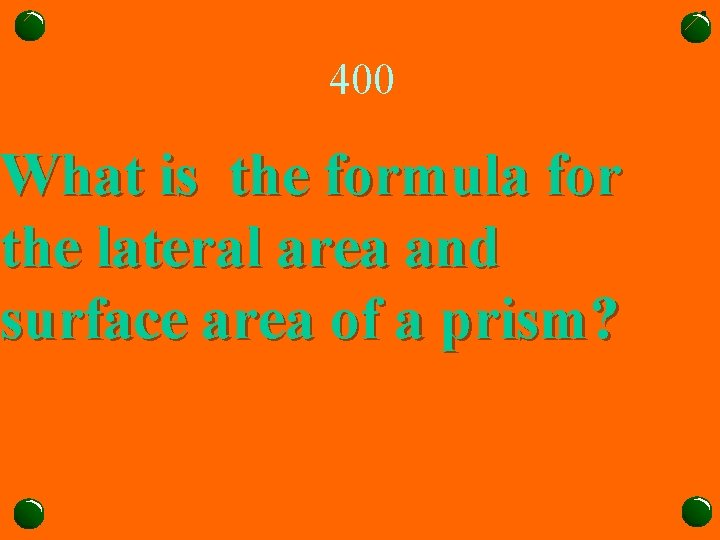400 What is the formula for the lateral area and surface area of a prism?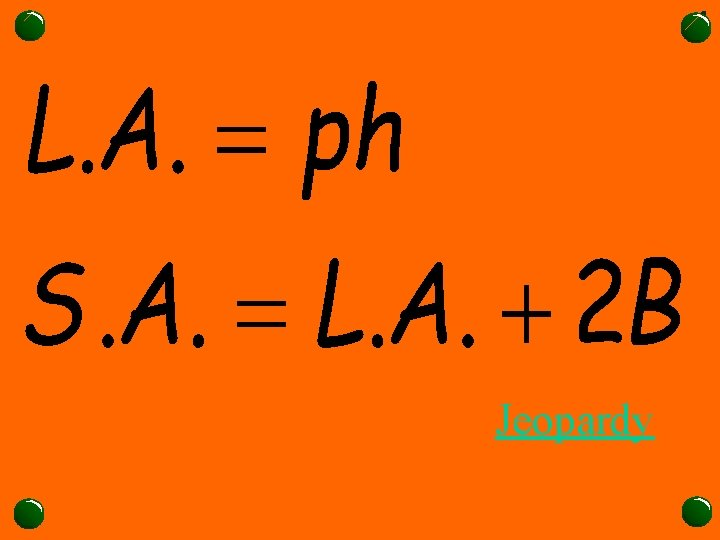Jeopardy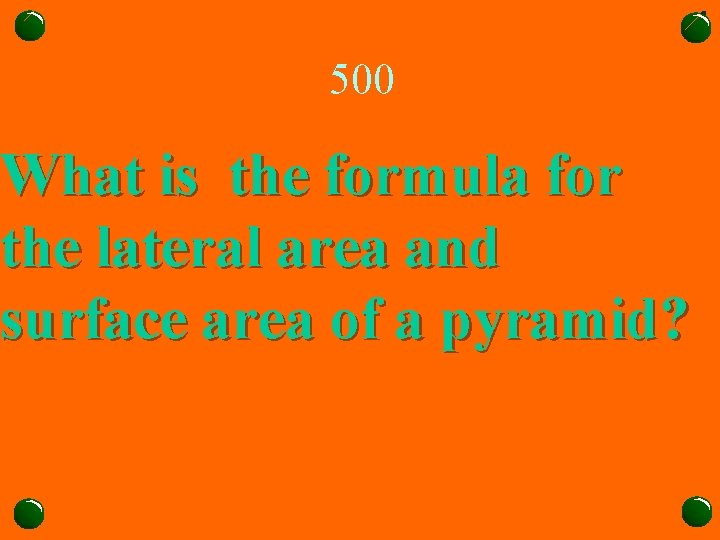500 What is the formula for the lateral area and surface area of a pyramid?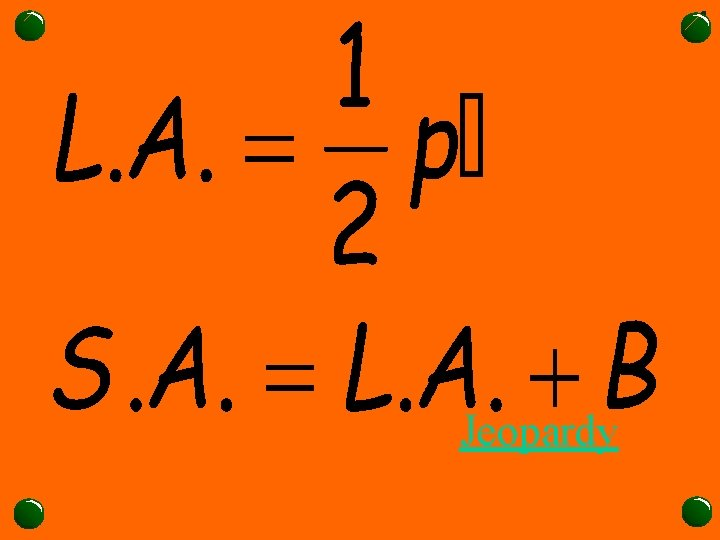Jeopardy